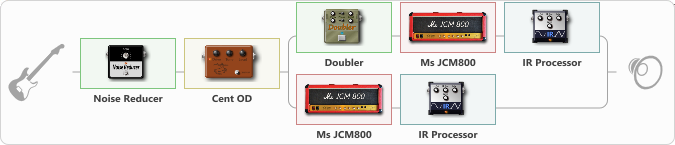# Anthrax: Amoung Rythm set

Discussion in 'ToneLib-GFX presets' started by BGelais, Nov 28, 2022.

1. Anthrax: Amoung Rythm set
Preset name: Anthrax Amoung The Living

Effects chain:Effect: "Noise Reducer" (Dynamics / Filter), active - "yes"
{

"Sens" = 90
"Mode" = Soft
}

Effect: "Cent OD" (Overdrive / Distortion), active - "yes"
{

"Drive" = 0
"Tone" = 76
"Level" = 100
}

Effect: "Splitter" (Dynamics / Filter)
{

"A-Bypass" = Off
"A-Pan" = -100
"A-Level" = 50
"B-Bypass" = Off
"B-Pan" = 100
"B-Level" = 50
"Width" = 100
'A' branch:
{

Effect: "Doubler" (Dynamics / Filter), active - "yes"
{

"A-Dly" = 75
"A-Inv" = Off
"A-Level" = 75
"B-Dly" = 50
"B-Inv" = Off
"B-Level" = 50
}
Effect: "Ms JCM800" (Amp simulators), active - "yes"
{

"Gain" = 100
"Bass" = 100
"Middle" = 0
"Treble" = 50
"Presence" = 0
"Master" = 32
"Level (dB)" = 0
}
Effect: "IR Processor" (Cabinets), active - "yes"
{

"IR" = Anthrax-Mosh_IR
"Low Cut (Hz)" = 0
"Hi Cut (kHz)" = 20.0
"Mix" = 100
"Level (dB)" = 0
}
}
'B' branch:
{

Effect: "Ms JCM800" (Amp simulators), active - "yes"
{

"Gain" = 100
"Bass" = 100
"Middle" = 0
"Treble" = 50
"Presence" = 0
"Master" = 32
"Level (dB)" = 0
}
Effect: "IR Processor" (Cabinets), active - "yes"
{

"IR" = Anthrax-Mosh_IR
"Low Cut (Hz)" = 0
"Hi Cut (kHz)" = 20.0
"Mix" = 100
"Level (dB)" = 0
}
}
}

Note: You will need to download and install the ToneLib-GFX software to use the preset.

#### Attached Files:

• ###### Anthrax_Amoung_Rythm_set.tlgfx
File size:
6.8 KB
Views:
2,955
Last edited: Nov 29, 2022
lautaro likes this.